# dataPlotOptions

Option set for `idplot` when plotting input/output estimation data contained in a timetable, numeric matrices, or an `iddata` object

Since R2023a

## Syntax

``opt = dataPlotOptions('time')``
``opt = dataPlotOptions('frequency')``
``opt = dataPlotOptions(___,'identpref')``

## Description

example

````opt = dataPlotOptions('time')` creates the default option set for plotting time-domain data. Use dot notation to customize the option set, if needed.```

example

````opt = dataPlotOptions('frequency')` creates a default option set for plotting frequency-domain data. Use dot notation to customize the option set, if needed.```

example

````opt = dataPlotOptions(___,'identpref')` initializes the plot options with the System Identification Toolbox™ preferences. This syntax can include any of the input argument combinations in the previous syntaxes. Use this syntax to change a few plot options but otherwise use your toolbox preferences.```

## Examples

collapse all

Create an options set with default options for time-domain data.

`opt = dataPlotOptions('time');`

Specify plot properties, such as time units and grid. View the plot in minutes

``` opt.TimeUnits = 'minutes'; % Turn grid on opt.Grid = 'on';```

Create a plot using the specified options.

```load iddata1 z1 h = idplot(z1, opt);```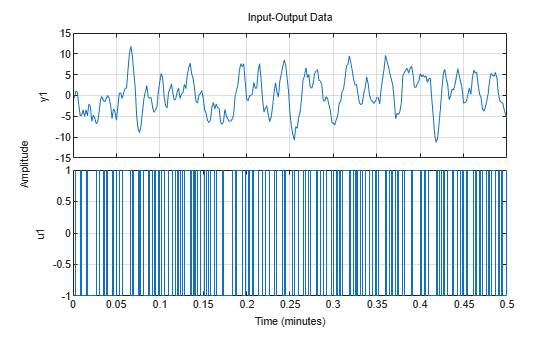Generate data with two inputs and one output.

`z = iddata(randn(100,1),rand(100,2));`

Configure a time plot.

`opt = iddataPlotOptions('time');`

Plot the data.

`h = plot(z,opt);`Change the orientation of the plots such that all inputs are plotted in one column, and all outputs are in a second column.

```opt.Orientation = 'two-column'; h = plot(z,opt);```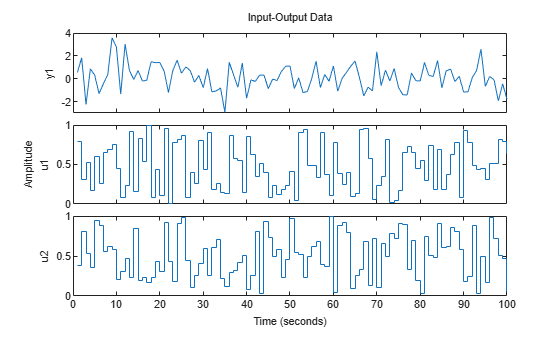Alternatively, use `setoptions`.

`setoptions(h,'Orientation','two-column')`

You can also change the orientation by right-clicking the plot and choosing `Orientation` in the context menu.

Create an option set with default options for frequency-domain data.

`opt = dataPlotOptions('frequency');`

Specify plot properties, such as phase visibility and frequency units.

```opt.PhaseVisible = 'off'; opt.FreqUnits = 'Hz';```

Create a plot with the specified options.

```load iddata7 z7 zf = fft(z7); h = idplot(zf,opt);```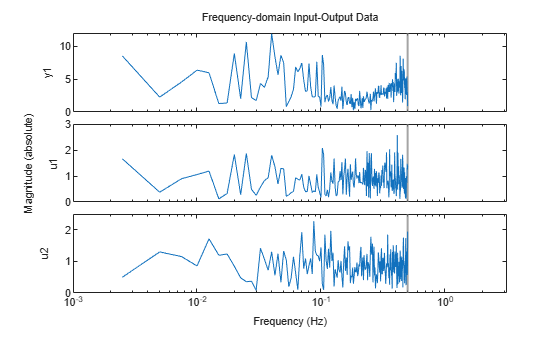`opt = dataPlotOptions('time','identpref');`

## Output Arguments

collapse all

Option set containing the specified options for `plot`. The structure has the following fields:

Field Description
`Title`, `XLabel`, `YLabel`

Text and style for axes labels and plot title, specified as a structure array with the following fields: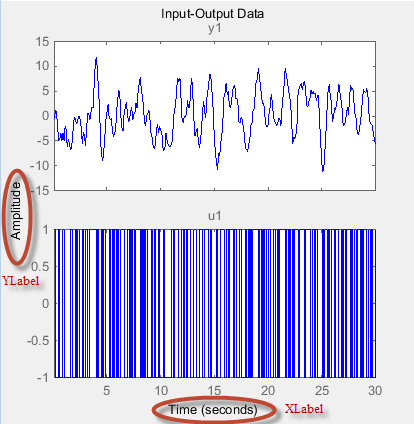• `String` — Title and axes label text, specified as a character vector.

Default `Title`: `'Input-Output Data'`

Default `XLabel`: `'Time'`

Default `YLabel`: `'Amplitude'`

• `FontSize` — Font size, specified as scalar value greater than `0`.
Default: `8`

• `FontWeight` — Thickness of text, specified as one of the following values: `'Normal'` | `'Bold'`
Default: `'Normal'`

• `Font Angle` — Text character angle, specified as one of the following values: `'Normal'` | `'Italic'`
Default: `'Normal'`

• `Color` — Color of text, specified as vector of RGB values between `0` to `1`.
Default: `[0,0,0]`

• `Interpreter` — Interpretation of text characters, specified as one of the following values: `'tex'` | `'latex'`| `'none'`
Default: `'tex'`

`TickLabel`

Tick label style, specified as a structure array with the following fields:

• `FontSize` — Font size, specified as scalar value greater than `0`.
Default: `8`

• `FontWeight` — Thickness of text, specified as one of the following values: `'Normal'` | `'Bold'`
Default: `'Normal'`

• `Font Angle` — Text character angle, specified as one of the following values: `'Normal'` | `'Italic'`
Default: `'Normal'`

• `Color` — Color of text, specified as vector of RGB values between `0` to `1` | character vector of color name | `'none'`. For example, for yellow color, specify as one of the following: `[1 1 0]`, `'yellow'`, or `'y'`.
Default: `[0,0,0]`

`Grid`

Show or hide the grid, specified as one of the following values: `'off'` | `'on'`

Default: `'off'`

`GridColor`

Color of the grid lines, specified as one of the following values: vector of RGB values in the range `[0,1]` | character vector of color name | `'none'`. For example, for yellow color, specify as one of the following: `[1 1 0]`, `'yellow'`, or `'y'`.

Default: `[0.15,0.15,0.15]`

`XlimMode`, `YlimMode`

Axes limit modes, specified as one of the following values:

• `'auto'` — The axis limits are based on the data plotted

• `'manual'` — The values explicitly set with `Xlim`, `Ylim`

Default: `'auto'`

`Xlim`, `Ylim`

Axes limits, specified as maximum and minimum values.

Default: ```[0 1]```

`IOGrouping`

Grouping of input-output pairs, specified as one of the following values: `'none'` | `'inputs'` | `'outputs'`|`'all'`

Default: `'none'`

`InputLabels`, `OutputLabels`

Input and output label styles on individual plot axes, specified as a structure array with the following fields: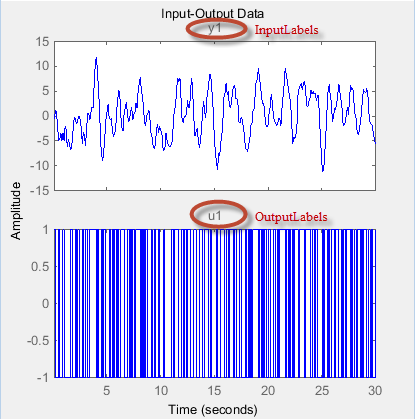• `FontSize` — Font size, specified as data type `scalar`.
Default: `8`

• `FontWeight` — Thickness of text, specified as one of the following values: `'Normal'` | `'Bold'`
Default: `'Normal'`

• `Font Angle` — Text character angle, specified as one of the following values: `'Normal'` | `'Italic'`
Default: `'Normal'`

• `Color` — Color of text, specified as a vector of RGB values between `0` to `1` | character vector of color name | `'none'`. For example, for yellow color, specify as one of the following: `[1 1 0]`, `'yellow'`, or `'y'`.
Default: `[0.4,0.4,0.4]`

• `Interpreter` — Interpretation of text characters, specified as one of the following values: `'tex'` | `'latex'`| `'none'`
Default: `'tex'`

`InputVisible`, `OutputVisible`

Visibility of input and output channels, specified as one of the following values: `'off'` | `'on'`

Default: `'on'`

`Orientation`

Orientation of the input and output data plots, specified as one of the following values:

• `'two-row'` — Plot all outputs in one row and all inputs in a second row

• `'two-column'` — Plot all outputs in one column and all inputs in a second column

• `'single-row'` — Plot all inputs and outputs in one row

• `'single-column'`— Plot all inputs and outputs in one column

Default: `'two-row'`.

For time-domain data plots only:

FieldDescription
`TimeUnits`

Time units, specified as one of the following values:

• `'nanoseconds'`

• `'microseconds'`

• `'milliseconds'`

• `'seconds'`

• `'minutes'`

• `'hours'`

• `'days'`

• `'weeks'`

• `'months'`

• `'years'`

You can also specify `'auto'` which uses time units specified in the `TimeUnit` property of the data. For multiple systems with different time units, the units of the first system is used.

`Normalize`

Normalize responses, specified as one of the following values: `'on'` |`'off'`

Default: `'off'`

For frequency-domain data plots only:

FieldDescription
`FreqUnits`

Frequency units, specified as one of the following values:

• `'Hz'`

• `'rad/second'`

• `'rpm'`

• `'kHz'`

• `'MHz'`

• `'GHz'`

• `'rad/nanosecond'`

• `'rad/microsecond'`

• `'rad/millisecond'`

• `'rad/minute'`

• `'rad/hour'`

• `'rad/day'`

• `'rad/week'`

• `'rad/month'`

• `'rad/year'`

• `'cycles/nanosecond'`

• `'cycles/microsecond'`

• `'cycles/millisecond'`

• `'cycles/hour'`

• `'cycles/day'`

• `'cycles/week'`

• `'cycles/month'`

• `'cycles/year'`

Default: `'rad/s'`

You can also specify `'auto'` which uses frequency units `rad/TimeUnit` relative to system time units specified in the `TimeUnit` property. For multiple systems with different time units, the units of the first system are used.

`FreqScale`

Frequency scale, specified as one of the following values: `'linear'` | `'log'`

Default: `'log'`

`MagUnits`

Magnitude units, specified as one of the following values: `'dB'` | `'abs'`

Default: `'dB'`

`MagScale`

Magnitude scale, specified as one of the following values: `'linear'` | `'log'`

Default: `'linear'`

`MagVisible`

Magnitude plot visibility, specified as one of the following values: `'on'` | `'off'`

Default: `'on'`

`MagLowerLimMode`

Enables a lower magnitude limit, specified as one of the following values: `'auto'` | `'manual'`

Default: `'auto'`

`MagLowerLim`Lower magnitude limit, , specified as data type `double`. It is typically decided by the range of the amplitudes the plotted data takes.
`PhaseUnits`

Phase units, specified as one of the following values: `'deg'` | `'rad'`

Default: `'deg'`

`PhaseVisible`

Phase plot visibility, specified as one of the following values: `'on'` | `'off'`

Default: `'on'`

`PhaseWrapping`

Enable phase wrapping, specified as one of the following values: `'on'` | `'off'`

Default: `'off'`

`PhaseWrappingBranch`

Phase value at which the plot wraps accumulated phase when `PhaseWrapping` is set to `'on'`.

Default: –180 (phase wraps into the interval [–180º,180º))

`PhaseMatching`

Enable phase matching, specified as one of the following values: `'on'` | `'off'`

Default: `'off'`

`PhaseMatchingFreq`Frequency for matching phase, specified as data type `double`.
`PhaseMatchingValue`The value to which phase responses are matched closely, specified as a real number representing the desired phase value `PhaseMatchingFreq`.

## Version History

Introduced in R2023a

expand all